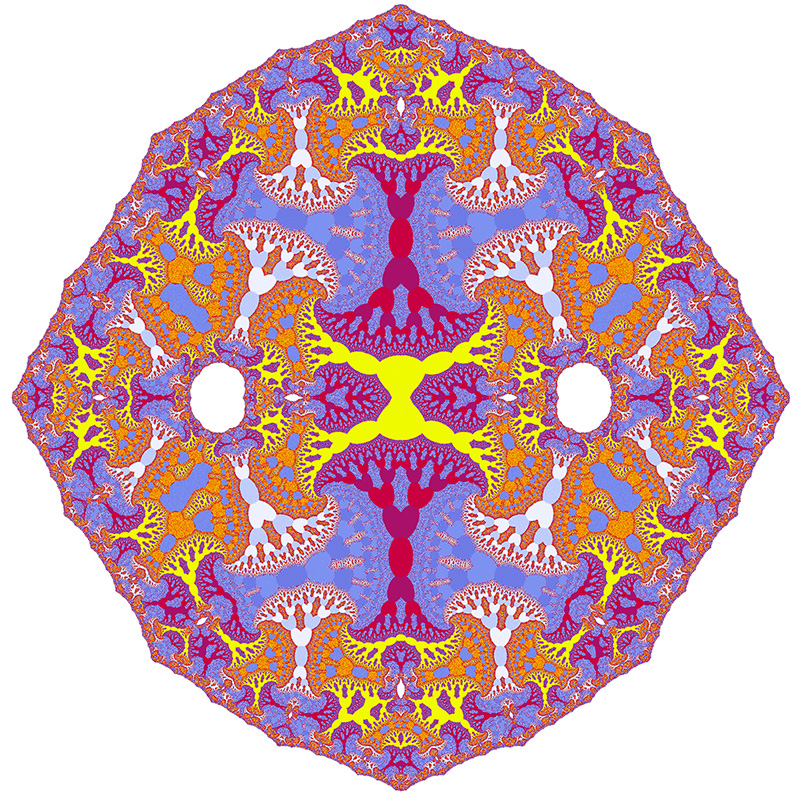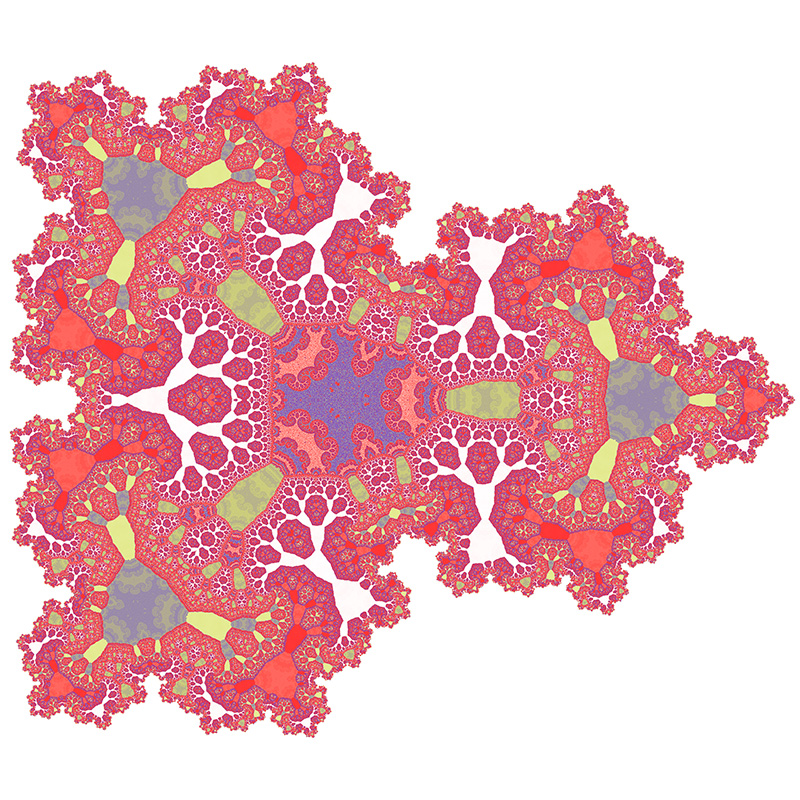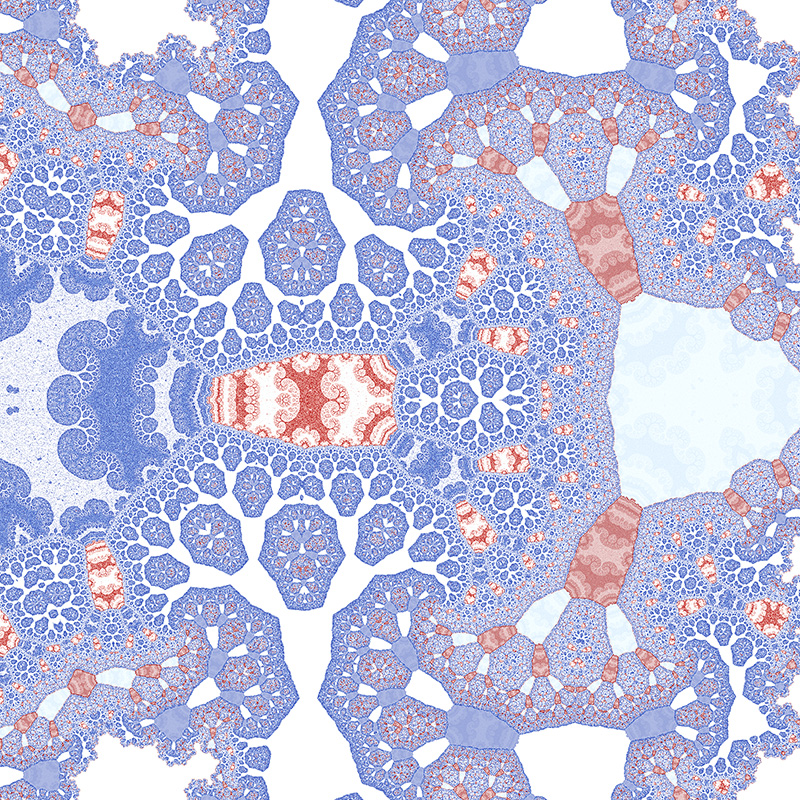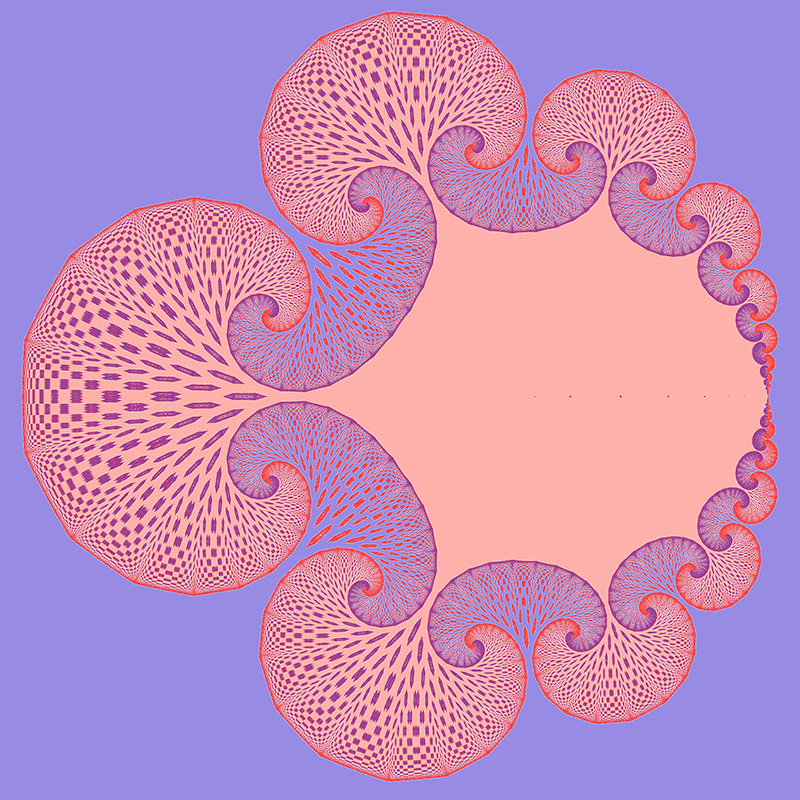# iabs fractal

Written by Paul Bourke
Inspired by Whittaker Courtney
July 2019

The following fractal is formed by considering the behaviour of the following complex series.

zn+1 = sinh(log(iabs((zn+c+1)*(zn+c-1))))

The iabs(z) function takes the absolute value of the imaginary component of z, leaving the real component unchanged. Where z0 is the point on the complex plane under consideration, and c a complex constant in this case equal to (0.555,0.15).zn+1 = iabs(zn1.3314+c)*iabs(zn1.3314-c)
where c = -0.818+0izn+1 = iabs(zn-0.555+c)*iabs(zn-0.555)
where c = -0.3+0i